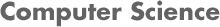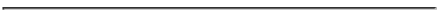### CS4341 Introduction to Artificial Intelligence Homework 3 and Sample Exam Problems A Term 2017

#### Prof. Carolina Ruiz and Ahmedul Kabir

Due Date: Canvas submission by Monday, Oct. 9th, 2017 at 11 am.This homework assignment consists of 2 parts:
1. Assigned problems: You need to submit your solutions on Canvas.
2. Sample exam problems: No need to submit your solutions but you should study them for Exam 3. Try to solve these problems first as this will help you with the HW problems too!

1. #### Assigned HW problems:

• Read Chapters/Sections 18.1-18.4, 18.9, 18.7, 22, 23 and 24 of the textbook.

• Problems: Submit your answers on Canvas to the problems below.

• Machine Learning - Chapter 18: (pp. 763-767)

1. Decision Trees:

1. Problem 1 from HW6 CS534 Spring 2013

2. 18.6 optional

2. Naive Bayes:

1. Use the the Naive Bayes network in the class handout to predict the Risk of the following data instance:
Credit History = bad; Debt = high; Collateral = adequate; and Income = >35. Show your work.

2. Problem 2 from HW6 CS534 Spring 2013

3. Artificial Neural Networks and Deep Learning:

1. Consider the error back propagation algorithm:
1. Is this algorithm a supervised or an unsupervised learning algorithm? Explain.
2. What type of search does it employ? Your answer here should be one specific search methods of those covered in project 1. Be precise.
3. What heuristic function does it use?
4. List 3 different possible termination conditions for the algorithm.

2. Investigate and explain the differences among the following types of "units" (i.e., perceptrons) in terms of the activation functions that they use. In addition, provide a graphical depiction of these activation functions. Are there some pairs on this list that are the same? Explain your answer.
• Linear
• Sigmoid
• ReLU
• SeLu
• Tanh

3. Explain the differences among the following types of layers in deep networks:
• Convolutional layer
• Fully connected layer
• Softmax layer

4. Define what an autoencoder is. Describe how an autoencoder is trained.

4. Evaluating machine learning models:
Assume that the following confusion matrix was obtained from using a machine learning model to predict the classifcation of 24 test instances, where the target attribute is Risk, with possible values low, moderate, and high:
```  a  b  c   <-- classified as
4  0  1 |  a = low
0  1  3 |  b = moderate
1  2 12 |  c = high
```
For each of the following metrics used in project 3, provide a formula that defines the metric, and calculate its value based on the confusion matrix above. Show your work.
1. The model's classification accuracy.
2. The model's classification error (= 100% - model's classification accuracy).
3. The model's precision for class=low.
4. The model's recall for class=moderate.

• Machine Vision - Chapter 24:

1. Briefly describe each of the following steps of the image understanding process. Try to write your answer in terms of what each step receives as its input and what it produces as its output:
• Segmentation
• Determining pose (position and orientation)
• Determining shape from contour, shading, texture, motion, and/or stereo vision
• Object recognition by matching against templates in a knowledge base

2. Briefly describe how deep learning - in particular convolutional neural networks and autoencoders - have been used for image understanding and object recognition.

• Natural Language Processing - Chapters 22 & 23:

1. Briefly describe each of the following steps of natural language understanding. Try to write your answer in terms of what each step receives as its input and what it produces as its output:
• Speach recognition
• Syntactic analysis
• Semantic analysis
• Pragmatic analysis

2. Briefly describe what natural language generation does and what its challenges are.

3. Statistical NLP: The following approaches explore statistical natural language processing, also known as text mining.

• k-gram Models: (See Section 22.1.1 of the textbook.) Use the "The Gift of the Magi" text (this is one of the most popular American short stories) to answer the following questions. Hint: Use the search feature of a text editor or a brower to count words quickly. Disregard lower/upper case differences and all punctuation.

1. A uni-gram (= 1-gram) word model of this text would determine the frequency with which each word appears in this text. Determine the frequency of just the following words in this text:
• the
• week
• gifts

2. A bi-gram (= 2-gram) word model of this text would determine the frequency with which each sequence of two consecutive words appears in this text. Determine the frequency of just the following bi-grams in this text:
• twenty dollars
• my hair
• brilliant sparkle

3. Just for fun, look at the to see the frequency of letters in the English language and the frequency of words in the English language.

• Text Classification / Categorization using Bag-of-Words: (See Section 22.1.4 of the textbook and some simple examples at http://datameetsmedia.com/bag-of-words-tf-idf-explained/)
In text classification, supervised machine learning is used to classify documents according to predefined categories (e.g., sports article, politics article, science article). A common approach is to convert text (unstructured data) to a bag-of-words (structured data). To do this, the following text preprocessing steps are used: (1) Tokenization: where words are extracted from sentences/text; (2) Stop word removal: where words like "a", "the", "with" are removed; and (3) Stemming: where related words are reduced to their "stem" form (e.g., "swim", "swam", "swims", "swimming" are all made equal to "swim").

Use the LAST PARAGRAPH in the "The Gift of the Magi" text only to answer the following questions. Disregard lower/upper case differences and all punctuation.

1. Which groups of words on that paragraph can be made equal using stemming?

2. After applying tokenization, stop word removal, and stemming, construct the bag-of-words for just that paragraph that counts the frequency of each (non-stop, stem) word in that paragraph.

• Deep Learning: Briefly describe how deep learning - in particular recurrent neural networks - have been used for natural language processing.

2. #### Additional sample exam problems:

Practice for the exam by solving the previous exams and homework problems from Prof. Ruiz's offerings of this course listed below. Solve the problems on your own before looking at the provided solutions. No need to submit your answers as part of your homework submission.